File: README.md Documentation text/markdown Documentation PHP Rectangle Class Perform operations and draw rectangle shapes By Win Aung Cho Update README.md Update README.md 7 months ago 3,533 bytes

## ContentsDownload

# PHP-RECTSLIB

This class Lib help you to construct the rectangles using various logical operations such as Union, Intersect and Subtract. It will generate the border edges of non-overlapped rectangles. JScript version

## Algorythm

Algorythm is very simple. - A region on which contains all the rectangles is divided into several rectangles according to the corners of the rectangles. - Remove the rectangles above which does not lie inside all rectangles. This step gives the non-overlapped union of the rectangles. - Then choose or remove the rectangles which lies inside the subject rectangles according to boolean operation union, intersect or subtract.

## Operation procedure

### Create rectangles

Rectangles are represenred by the associative array in which diagonal points p1 and p2 are stored. Other variables for drawing are name, color and state. Point p1 is always upper left corner and p2 is for lower right of rectangles so that p1 has smaller value of x and y then p2.

``````\$rectslib = new RectsLib();
\$A = \$rectslib->p1p2(10, 10, 300, 200, "A", \$green);
\$B = \$rectslib->p1p2(40, 30, 200, 300, "B", \$red);
\$C = \$rectslib->p1p2(150, 40, 400, 150, "C", \$orange);
\$D = \$rectslib->p1p2(140, 320, 200, 400, "D", \$blue);
\$E = \$rectslib->p1p2(80, 160, 250, 250, "E", \$brown);
\$rects = [\$A, \$B, \$C, \$D, \$E];
``````

### Merge all rectangles into mesh

List all x and y coordinates from the corner points p1, p2 of rectangles. Sort x and y list and then create rectangles for all x and y. Get (2n-1)^2 mesh rectangles for n original rectangles.

``````\$all = \$rectslib->merge(\$rects, \$grey);
``````

### Generate rectangle operation

Select mesh by checking inside or not the desired rectangles which for logical operation. Eg. If A B C D E are the recangles, - For union of [A B C] & [D E], mesh must be lied in side both of [A B C] & [D E]. - For subtract [B E] from [A B C D], mesh must be inside the [A B C D] and outside of [B E]. - For intersect [A B C D] & [B E], mesh must be inside of both [A B C] & [D E].

``````\$uni = \$rectslib->union([\$A, \$B, \$C], [\$E, \$D]);
\$subt = \$rectslib->subtract([\$A, \$B, \$C, \$D], [\$B, \$E]);
\$Intersect = \$rectslib->intersect([\$A, \$B, \$C, \$D], [\$B, \$E]);
``````

### Generate bounding edges

Generate edges of each rectangles and select external edges by removing common edges.

``````\$edges = \$rectslib->genEdges(\$subt);
\$rectslib->drawEdges(\$img, \$edges, \$white);
``````

## Check with Images

### Original Rectangles

• A-GREEN-{10,10:300,200}
• B-RED-{40,30:200,300}
• C-ORANGE-{150,40:400,150}
• D-BLUE-{140,320:200,400}
• E-BROWN-{80,160:250,250}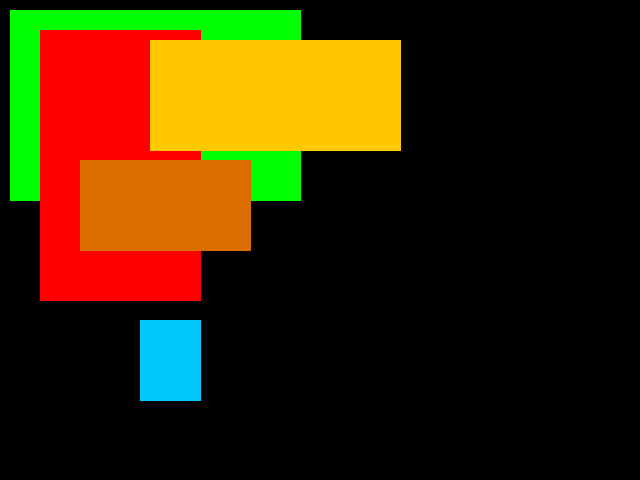### Start meshing for all rectangles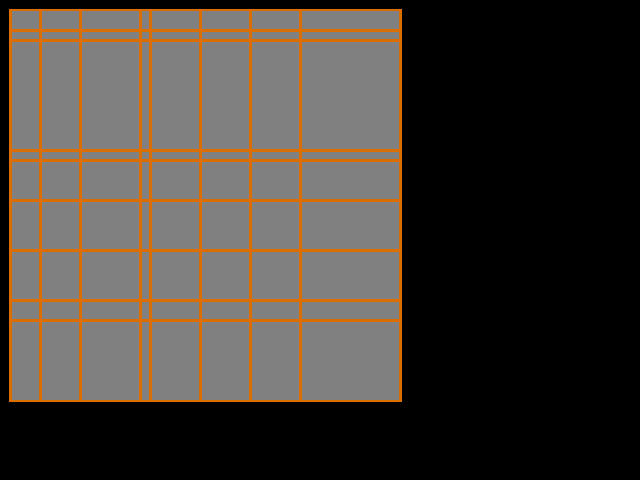### Meshing and get union of rectangles

• Union of [A B] & [C D]
• [A B] ? [C D]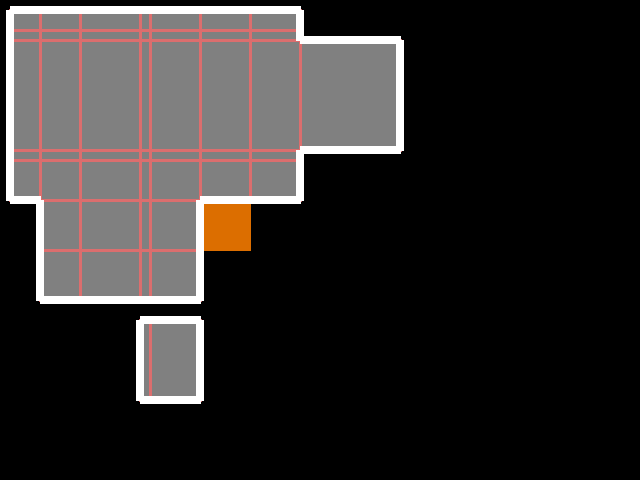### Intersection of all with 2 rectangles

• Intersect of [A B C D] & [B E]
• [A B C D] ? [B E]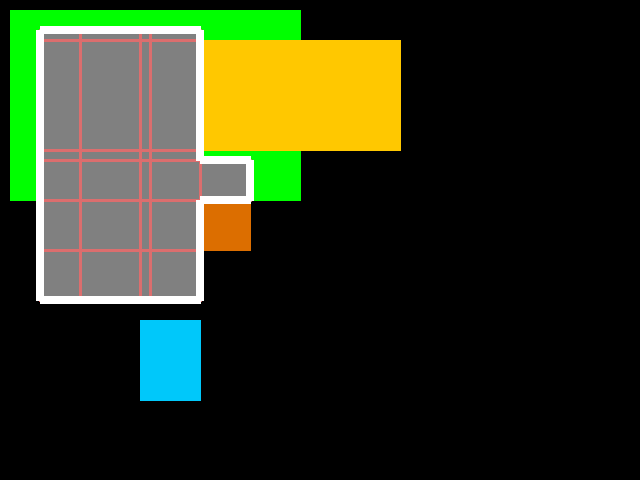### Subtract of 2 from all

• Subtract [B E] from [A B C D]
• [A B C D] - [B E]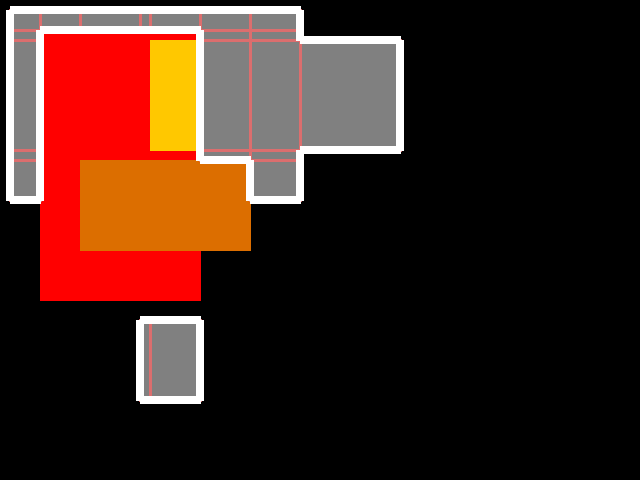## Contact

Contact me for comercial use via mail winaungcho@gmail.com.About usAdvertise on this siteSite mapNewsletterStatisticsSite tipsPrivacy policyContact
For more information send a message to `info at phpclasses dot org`.